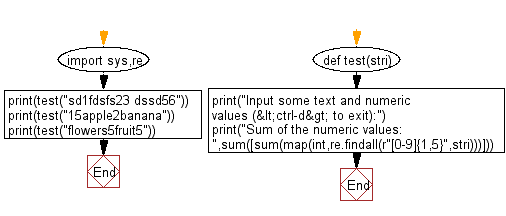﻿ Python: Sum of all numerical values embedded in a sentence - w3resource# Python: Sum of all numerical values embedded in a sentence

## Python Basic - 1: Exercise-56 with Solution

Write a Python program to sum of all numerical values (positive integers) embedded in a sentence.

Input:
Sentences with positive integers are given over multiple lines. Each line is a character string containing one-byte alphanumeric characters, symbols, spaces, or an empty line. However the input is 80 characters or less per line and the sum is 10,000 or less.

Sample Solution:

Python Code:

``````import sys,re
def test(stri):
print("Input some text and numeric values (<ctrl-d> to exit):")
print("Sum of the numeric values: ",sum([sum(map(int,re.findall(r"[0-9]{1,5}",stri)))]))

print(test("sd1fdsfs23 dssd56"))
print(test("15apple2banana"))
print(test("flowers5fruit5"))
``````

Sample Output:

```Input some text and numeric values ( to exit):
Sum of the numeric values:  80
None
Input some text and numeric values ( to exit):
Sum of the numeric values:  17
None
Input some text and numeric values ( to exit):
Sum of the numeric values:  10
None
```

Pictorial Presentation:Flowchart:Python Code Editor:

Have another way to solve this solution? Contribute your code (and comments) through Disqus.

What is the difficulty level of this exercise?

Test your Programming skills with w3resource's quiz.

﻿

## Python: Tips of the Day

Iterating over dictionaries using 'for' loops:

I am a bit puzzled by the following code: d = {'x': 1, 'y': 2, 'z': 3} for key in d: print key, 'corresponds to', d[key] What I don't understand is the key portion. How does Python recognize ...

key is just a variable name.

```for key in d:
```

For Python 3.x:

```for key, value in d.items():
```

For Python 2.x:

```for key, value in d.iteritems():
```

To test for yourself, change the word key to poop.

In Python 3.x, iteritems() was replaced with simply items(), which returns a set-like view backed by the dict, like iteritems() but even better. This is also available in 2.7 as viewitems().

The operation items() will work for both 2 and 3, but in 2 it will return a list of the dictionary's (key, value) pairs, which will not reflect changes to the dict that happen after the items() call. If you want the 2.x behavior in 3.x, you can call list(d.items()).

Ref: https://bit.ly/37dm0Qo